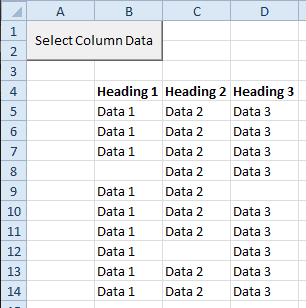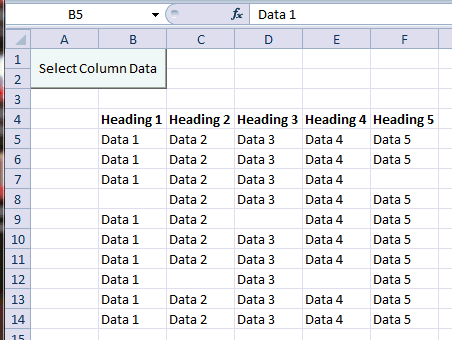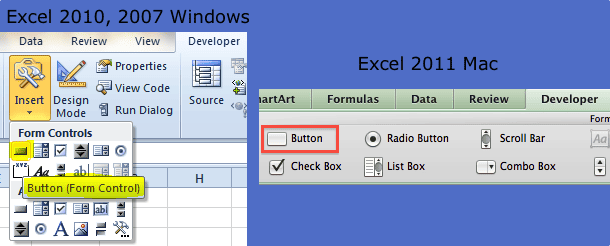# Select One Column of Data with VBA

Let’s assume you aren’t using an Excel Table, List Object, or List for your data table and you want to select the data in only one column, which means the Header row is excluded. This can be simple if there’s data in every row of the column, but what if that’s not the case?And assume for the moment that the example above extends down several hundred, or even thousand rows. I would get tired of manually selecting the data in a column by using the Ctrl+Shift+down arrow keyboard shortcut.

So I’d probably write some VBA code to handle this pesky task and link it to a button on the worksheet. Below is the VBA code.
``` Sub SelectColumnData() Dim ac As Range Dim cr As Range Dim col As Integer```

``` Set ac = ActiveCell Set cr = ac.CurrentRegion col = ac.Column - cr.Column ```

``` If cr.Rows.Count > 1 Then cr.Offset(1, col).Resize(cr.Rows.Count - 1, 1).Select End If End Sub ```

The active cell must be in the column you want to select when running this macro.To insert the button in Excel 2011, 2007 (Windows), I’m using a Form control by choosing Developer > Insert, selecting the Button icon, and drawing a button on the worksheet with the help of the Alt key to snap to cell borders. In Excel 2011 (Mac) choose Developer on the Ribbon then click the Button icon and draw a button on the worksheet. The alt/control key doesn’t work to snap to gridlines.Then I rename the button and assign the macro.I can also assign a shortcut to the Macro instead of using a button, but the only issue with that is remembering what the shortcut is when I need to use the macro.This entry was posted in Advanced and tagged on by .

## 5 thoughts on “Select One Column of Data with VBA”

1.Ab

Great tip! exactly what I was looking for

2.Bob

This macro also works for Excel-2003 (Windows).

Thank you Gregory — this is a real time-saver.

1.Gregory

@Bob, I’m glad you think it’s useful, I kind of like it myself.

3.Pavel

Hi Gregory,
What is I have data like this:

7

13
11

13
11

19
17
11

23
17

and I want select it all? How should I change the macro code?
Thanks

1.Gregory

If you put a heading above the first numerical entry, this code will select the column of data.

``` Sub SelectOneColumnData() ''''''''''''''''''''''''''''''''''''''''''''''''''''''''''''''' ' This routine will select a non-continuous column of data ' when active cell is located in the column heading. ''''''''''''''''''''''''''''''''''''''''''''''''''''''''''''''' Dim ac As Range Dim ws As Worksheet Dim lRow As Long Dim lc As Range Dim col As Integer Dim cr As Range```

``` ' Set active cell and row variables Set ac = ActiveCell col = ac.Column ' Get the last row of the worksheet, then ' set the last row in the current column lRow = ActiveSheet.Rows.Count Set lc = Cells(lRow, col) ' Find the bottom of the range in the current column, then ' re-set the last cell range Set lc = lc.End(xlUp) lRow = lc.Row ```

```' Set the current range from the active cell to the last row ' in the column with data Set cr = ac.Offset(1, 0).Resize(lc.Row - ac.Row, 1) cr.Select End Sub ```